# Guest post by Amir Ali Ahmadi: Sum of Squares (SOS) Techniques: An Introduction, Part I/II

Sum of squares optimization is an active area of research at the interface of algorithmic algebra and convex optimization. Over the last decade, it has made significant impact on both discrete and continuous optimization, as well as several other disciplines, notably control theory. A particularly exciting aspect of this research area is that it leverages classical results from real algebraic geometry, some dating back to prominent mathematicians like Hilbert. Yet, it offers a modern, algorithmic viewpoint on these concepts, which is amenable to computation and deeply rooted in semidefinite programming. In this post, we give an introduction to sum of squares optimization focusing as much as possible on aspects relevant to ORF523, namely, complexity and interplay with convex optimization. A presentation of this length is naturally incomplete. The interested reader is referred to a very nice and recent edited volume by Blekherman, Parrilo, and Thomas, the PhD thesis of Parrilo or his original paper, the independent papers by Lasserre and by Nesterov, the paper by Shor (translated from Russian), and the survey papers by Laurent and by Reznick. Much of the material below can be found in these references.

Polynomial Optimization

For the purposes of this post, we motivate the sum of squares machinery through the polynomial optimization problem:

(1)where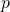,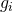, and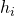are multivariate polynomials. A set defined by a finite number of polynomial inequalities (such as the setabove) is called basic semialgebraic. Of course, we can writewith polynomial inequalities only (by replacingwithand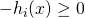), or (unlike the case of linear programming) with polynomial equalities only (by replacing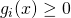with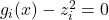, for some new variables). We prefer, however, to keep the general form above since we will later treat polynomial inequalities and equalities slightly differently.

It follows from the quantifier elimination theory of Tarski and Seidenberg that (the decision version of) problem (1) is decidable in its greatest generality. In other words, we can test in finite time (on a Turing Machine) whether a set of polynomial inequalities and equalities are feasible. This is in sharp contrast to problem (1), appended with integer constraints, which is undecidable even when the polynomials have fixed degree and dimension.

The special case of problem (1) where the polynomialsall have degree one is of course linear programming, which we can solve in polynomial time. Unfortunately though, as we will see in the complexity section of this post below, the problem quickly becomes intractable when the degrees increase from one ever so slightly. For example, unconstrained minimization of a quartic polynomial, minimization of a cubic polynomial over the sphere, or minimization of a quadratic polynomial over the simplex are all NP-hard.

The sum of squares methodology offers a hierarchy of polynomially sized semidefinite programming relaxations to cope with this computational intractability. It is quite different in philosophy from the approach taken by, say, the descent methods in nonlinear optimization. In particular, it makes absolutely no assumptions about convexity of the objective function, or the constraint set. Nevertheless, the hierarchy has a proof of asymptotic convergence to a globally optimal solution and in practice often the first few levels of the hierarchy suffice to solve the problem globally.

If we could optimize over nonnegative polynomials…

A point of departure for the sum of squares methodology is the observation that if we could optimize over the set of polynomials that take nonnegative values over given basic semialgebraic sets, then we could solve problem (1) globally. To see this, note that the optimal value of problem (1) is equal to the optimal value of the following problem:

(2)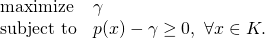Here, we are trying to find the largest constantsuch thatis nonnegative on the set. This formulation suggests the need to think about a few fundamental questions: given a basic semialgebraic setas in (1), what is the structure of the set of polynomials (of, say, some fixed degree) that take only nonnegative values on? Can we efficiently optimize a linear functional over the set of such polynomials? Can we even test membership to this set efficiently?

Observe that independent of the convexity of the set, the set of polynomials that take nonnegative values on it form a convex set! Albeit, as we see next, this convex set is not quite tractable to work with.

Complexity considerations

We first show that testing membership to the set of polynomials that take nonnegative values over a basic semialgebraic setis NP-hard, even when. In order to give a very simple reduction “from scratch”, we first prove this claim with the word “nonnegative” replaced by “positive”.

Theorem
Given a polynomialof degree, it is strongly NP-hard to decide if it is positive definite, i.e., iffor all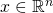.

Proof: Recall the NP-complete problem of PARTITION problem from Seb’s earlier lecture: Given integers, decide if there is a way to split them into two bags such that the sum of the integers in one bag equals the sum of the integers in the other. It is easy to see that a PARTITION instance is infeasible if and only if the quartic polynomialis positive definite (why?).

This already implies weak NP-hardness. Recall also from Seb’s lecture that PARTITION admits a simple pseudo-polynomial time algorithm based on dynamic programming. To obtain a strong NP-hardness result and rule out the possibility of a pseudo-polynomial time algorithm (unless P=NP), we give a slightly more involved reduction from ONE-IN-THREE 3SAT, taken from this paper. ONE-IN-THREE 3SAT is a close variant of 3SAT, which just like 3SAT is NP-hard in the strong sense. (The reason why we pick this problem over the more familiar 3SAT is that an equally straightforward reduction from the latter problem would only prove hardness of positivity testing for polynomials of degree.) In ONE-IN-THREE 3SAT, we are given a 3SAT instance (i.e., a collection of clauses, where each clause consists of exactly three literals, and each literal is either a variable or its negation) and we are asked to decide whether there exists aassignment to the variables that makes the expression true with the additional property that each clause has exactly one true literal.

To avoid introducing unnecessary notation, we present the reduction on a specific instance. The pattern will make it obvious that the general construction is no different. Given an instance of ONE-IN-THREE 3SAT, such as the following

(3)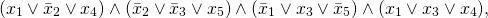we define the quartic polynomialas follows:

(4)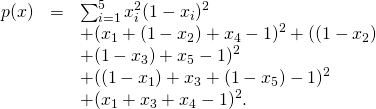Having done so, our claim is thatfor all(or generally for all) if and only if the ONE-IN-THREE 3SAT instance is not satisfiable. Note thatis a sum of squares and therefore nonnegative. The only possible locations for zeros ofare by construction among the points in. If there is a satisfying Boolean assignmentto (3) with exactly one true literal per clause, thenwill vanish at point. Conversely, if there are no such satisfying assignments, then for any point in, at least one of the terms in (4) will be positive and hencewill have no zeros.Deciding if a polynomialis nonnegative—i.e., iffor all—is also strongly NP-hard if we consider polynomials of degreeor higher even degree. A simple reduction is from the matrix copositivity problem: Given a symmetric matrix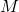, decide if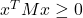for all. (Note the similarity to testing matrix positive semidefiniteness, yet the drastic difference in complexity.) To see the connection to polynomial nonnegativity, observe that the quartic homogeneous polynomial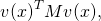with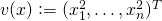, is nonnegative if and only ifis a copositive matrix.

Matrix copositivity arises in surprisingly many areas of optimization and more broadly computational mathematics. Its strong NP-hardness follows e.g. via a reduction from the STABLE SET problem (a.k.a. the INDSET problem), which can be found here. The main ingredient is a beautiful theorem of Motzkin and Straus (1965): The stability numberof a graphwith adjacency matrixsatisfiesA quadratic programming formulation makes sum of squares techniques directly applicable to the STABLE SET problem, and in a similar vein, applicable to any NP-complete problem. We end our complexity discussion with a few remarks.

1.  The set of nonnegative polynomials and the set of copositive matrices are both examples of convex sets for which optimizing a linear functional, or even testing membership, is strongly NP-hard. This observation deserves some attention in view of the common misconception about “convex problems being easy.” Seb already placed a word of caution about this in his very first lecture, where he showed that practically any optimization problem can be “written” as a convex problem. So the algebraic/geometric structure of the set, beyond convexity, cannot be ignored.
2. Back to the polynomial optimization problem in (1), the reductions we gave above already imply that unconstrained minimization of a quartic polynomial is NP-hard. The aforementioned hardness of minimizing a quadratic form over the standard simplex follows e.g. from the Motzkin-Straus theorem above. Unlike the case of the simplex, minimizing a quadratic form over the unit sphere is easy. We have seen in a previous lecture already that this problem (although non-convex in this formulation!) is simply an eigenvalue problem. On the other hand, minimizing forms of degree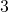over the unit sphere is NP-hard, due to a result of Nesterov.
3. Finally, we remark that for neither the nonnegativity problem nor the positivity problem did we claim membership in the class NP or co-NP. This is because these problems are still open! One may think at first glance that both problems should be in co-NP: If a polynomial has a zero or goes negative, simply present the vectorat which this happens as a certificate. The problem with this approach is that there are quartic polynomials, such as the following,for which the only zero takes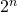bits to write down. Membership of these two problems in the class NP is much more unlikely. Afterall, how would you give a certificate that a polynomial is nonnegative?

To be continued…

This entry was posted in Optimization. Bookmark the permalink.